Antenna Factor Calculations and Deviations

or access our simple Antenna Factor and Gain Calculator

Introduction

In recent years, the use of a term call "Antenna Factor" in EMC and spectrum pollution work has become very important. There has been a great need for measuring field intensity and/or power density as accurately and conveniently as possible.

Classically in RFI/EMI measurements, the use use of antenna factors is very common. These antenna factors were, by definition, the conversion of two-terminal receiver voltages to field intensity. This is a very convenient term, particularly when expressed in decibels, and the receiver measures in dB above 1µ volt. Historically, antenna factors were supplied by equipment manufactures, or in some cases, designated in military specifications. In many cases, these factors were used incorrectly because the user did not understand fully the mathematical significance of the antenna factor. It is the purpose of this paper to establish the relationship of antenna factor and antenna gain.

Antenna gain is a common term utilized by antenna design engineers as well as communication electronic system designers the gain is the ratio of the power density produced by antenna at a certain range in a certain direction to the average power density at that range.

The recent deployment of computer controlled receiving systems with analysis capable capabilities has added a new need for examining the antenna Factor the desired data output from such systems is usually a field intensity Express involve per meter as a function of frequency. From the standpoint of strictly application of antenna Factors it would seem as easy test to store the antenna factor has a look up table in the computers core memory power as we shall see I may not necessary be the most cost effective or the best utilization of computer memory.

ANTENNA FACTOR CALCULATIONS

Antenna Factor FA is used in EMC/EMI testing to convert received voltage VR to the radiated field intensity ED. Depending on the type of test that antenna may include factors such as:
• Loss due to mismatch of impedance between the antenna output and transmission line.
• Loss due to attenuation of the transmission line.
• Loss due to VSWR at the and/or the receiver.
• Gain due to a preamplifier located at the antenna.
• Loss due to the mismatch of impedance at the input of the receiver

Although all of the above factors are very real losses of received signal, they may be treated separately and independently. They may be calculated, measured, or obtained from published charts. If any of the above factors are included in an antenna factor, it should be stated so as to avoid misinterpretation.

Let us assume a condition where the antenna is so designed that the above five factors are negligible, that is, well-matched antenna output/cable/receiver, short low loss cable, no preamp gain, low VSWR.

From the radio engineers handbook, the rated power density is: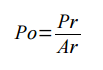1
where:
PO = Power Density
PR = Receiver Power
AR = Effective area antenna

However,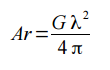2
where:
G = Antenna gain(numeric)
λ = Wavelength

Combining Eqs. 1 and 2:3

Again, from the radio engineers handbook: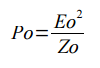4
EO = Field intensity
ZO = Impedance of radiating media

The power at the receiver is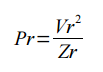5
where:
Vr = Voltage at the receiver
Zr = Receiver input impedance

Substituting the expressions for PO and PR of equations 4 and 5, respectively, into equation 3 yield: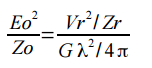6

Rearranging terms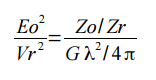7

Converting to decibels:89

By definition, the ratio (expressed in dB) of the field intensity to the received voltage is the antenna factor FA . Therefore,10

Equation 10 is the general form of the antenna factor as a function of source and load impedance, and antenna gain.

Many practical applications of antenna factors are in "free space" environment. This is, in general, true for antennas used outside which are directive and are not pointed with low grazing angles.

When ground effects are to be considered, the source impedance Zo must be modified.

For free space (377 ohm) and a 50-ohm receiving system, we have the impedance ratio expressed in dB as follows:11

And 4π expressed in decibels is as follows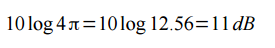12

Substituting into equation 10 the expressions obtained in equations 11 and 12, we have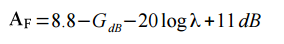1314

Equation 14 is the more useful form of the antenna factor which is a function of antenna gain and wavelength.

Many EMC engineers prefer to use frequency rather than wavelength because the measurement instrumentation is calibrated in frequency.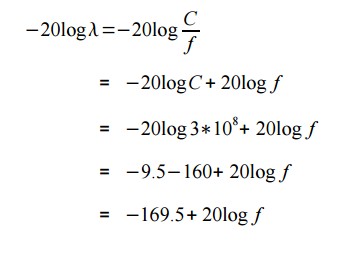15
Where
C = Propagation velocity

Substituting the results of equation 15 into equation 14 we have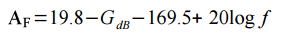16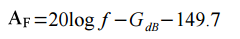17

Equation 17 is the reduced form of antenna factor for an ideal antenna as a function of antenna gain and frequency.

APPLICATIONS TO COMPUTER PROCESSING
If you want to calculate and display field intensity from a real-time data collection system, it is important to have the value for antenna factor available in the computer for field intensity calculations. Since the receivers in the system are controlled by the computer, the frequency is always known. If we examine equation 17, we see that if the receiving antenna were designed to have a constant gain over a band width of interest the only variable would be frequency. Thus, by utilizing a constant gain antenna, no lookup table is required and the computer simply makes three additional additions to compute field intensity. The result is that practically no computer cord is utilized and a calculation is simple

Other Resources:

Antenna Factor and Gain Calculations

Contact Details

A.H. Systems, inc.
9710 Cozycroft Ave.
Chatsworth, CA 91311

P: (818) 998-0223

F: (818) 998-6892

E: Email

Product Showcase

SAS-510-2
• 290 MHz to 2 GHz
• Compact, Lightweight Antenna
• High Power Input Capability

This directional Log Periodic Antenna is an ideal solution for radiated emissions and normalized site attenuation.

Have a gain value but need the Antenna Factor? or the other way around? This calculator will calculate the Antenna Factor (dB/m), gain (dBi), and gain (Numeric) values... [more...]

Product Showcase

Octave Horn Antennas:• 1 GHz - 8 GHz
• Linearly polarized
• Transmit and recieve

These Octave Horn Antennas offers excellent half power performance With low VSWR, high input handling capability and rugged design; excellent for both immunity and emissions testing.